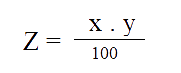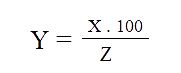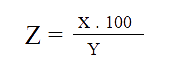# Percentage calculator

#### What is x % of y ? (Eg: 5% of 100 equal 5)

Formula:#### x is what % of y ? (Eg: 5 is 5% of 100 )

Formula:#### x is y% of what? (Eg: 5 is 1% of 500 )

Formula:A2Z tools provide free tools to calculate percentages.Tools are designed by our professional experts.It can calculate both simple and difficult calculations.

## What Is a Percentage Calculator?

A percentage calculator is a mathematical tool that can be used to calculate percentages. It is a number or ratio expressed in terms of hundred. A percentage is represented by %. The Percentage calculator allows you to input a number and it will output the percentage as a decimal. Find percentage quickly and accurate results. An increase or decrease in number can be determined by Percentage.

For Example:the price of the Mobile has increased by 30%, the price of the TV has decreased by 30% in the last few years, etc..

## Basic Percentage calculations

“X” percent of “Y”

“X” as a percentage of “Y”

Enter the numbers in the respective field

X% of Y ? (Eg. 5% of 100 equal 5)

Assume X is “5” and y is “100”, percentage formula is

5 is divided by 100 and multiplied by 100

### Calculate Percentage using Percentage formula

Percentage = (Value/Total value) x100

Let value = x and total value = y

Assume percentage as “Z”

So , Z = X * Y/100,

For Example:Z = 5*100/100 = 5.00

Result 5% of 100 equal 5

X is Y% of what ?

Percentage, % = (Value/Total value) x100

For Example:5 is 1% of 500

Formula is Z = X*100/Y = 5*100/1

Result 5 is 1% of 500

1. The percentage calculator helps in determining the profit or loss suffered by the business or companies.
2. percentage formula is used to promote the business by offering discounts in percentage.
3. Used For calculation of investment and loans in percentage.
4. Free tool, easy calculation, and user-friendly.

### What is the Percentage?

Percent is a Latin word for “ by hundred”.It means per hundred
Eg:1/100

Yes It's free